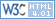Home Nav. course Sailing Greece Turkish Coasts Yacht charter Gulets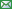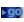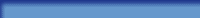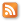## Doubled angle fix

The Doubled angle on the bow fix resembles a running fix though only one navigation aid is used.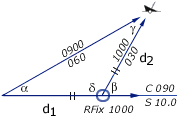α = 30° , β = 60°δ = 120° , γ = 30°Isosceles    d1 = d2
In the example on the right the initial angle (30°) on the bow is doubled (60°) yielding an isosceles triangle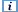. The distance travelled between the bearings is the same as the distance from the visible wreck.
• Start with the visible wreck having a bearing of less than 45° off the bow (α), note the log distance.
• Proceed along the course until the angle on the bow is doubled (β), read the log: d1 is 10 nm.
• Use the log distance to find the position on the second LOP. It is an isosceles triangle, so d2 is also 10 nm.
• Label it with an ellipse and "RFix" but realize it is less precise than a running fix that involves two navigation aids.

## Four point fix

If the first angle on the bow is 45°, a special situation occurs: The Four point fix, so called since 45 degrees equals 4 points on the compass (1 point = 11,25°).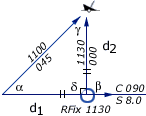α = 45° , β = 90°δ = 90° , γ = 45°Isosceles    d1 = d2
• Start with a bearing with 45° on the bow (α), note the log.
• Proceed along the course till the angle on the bow is 90° (β), read the log: d1 is 4 nm
• Use the log distance to find the position on the second LOP. Isosceles, so d2 is also 4 nm.
• Label it with an ellipse and "RFix".

## Special angle fix

The Special angle fix requires the mariner to know some special pairs of angles (a : b) that give the distance travelled between bearings as equal to the distance abeam.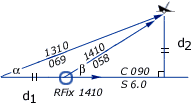α = 21° , β = 32°d1 = d2
In the example on the right α = 21° and β = 32° are used. Now, the log distance equals the shortest distance between wreck and course line (6 nm).
A few practical pairs:
16 : 22      21 : 32
25 : 41      32 : 59
37 : 72      40 : 79

Remember: the greater the angular spread the better. Hence, of these three fixes the four point fix is the most precise one.
 Enter α (1-45°): β:
Mathematics: isosceles triangle fixes

## Distance of the horizon

On a flat world there would be no difference between the visible and sensible horizon. However, on Earth the visible horizon appears several arc minutes below the sensible horizon due to two opposing effects: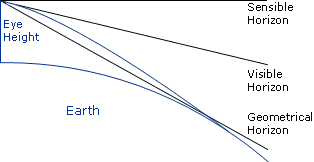• the curvature of the earth's surface;
• atmospheric refraction.

Atmospheric refrac­tion bends light rays pas­sing along the earth's sur­face to­ward the earth. There­fore, the geo­metr­ical hori­zon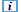ap­pears ele­vated, for­ming the vi­sible ho­rizon.
The distance of the visible horizon is a (semi-empirical) function of Eye Height: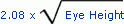Mathematics: horizon distances

## Dipping range

If an object is observed to be just rising above or just dipping below the visible horizon, its distance can be readily calculated using a simple formula.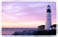The object's elevation (the height of a light above chart datum) can be found in the chart or other nautical publication such as the 'List of Lights'. Note that in some charts elevation is referred to a different datum than soundings. Click on the image on the right to view a magnificent lighthouse.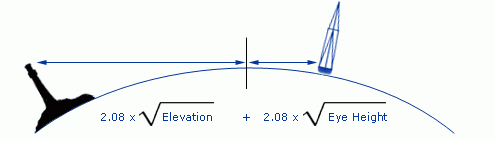The formula contains the two distances from the visible horizon and can be simplified by the equation: 2.08 x (√Elevation + √Eye height). Many nautical publications contain a table called "distances of the horizon" which can be used instead of the equation.
Use the dipping range to plot a Distance LOPin the chart: a circle equal in radius to the measured distance, which is plotted about the navigation aid. Finally, take a bearing on the object to get a second LOP and a position fix.
 Enter Eye height (metres): Enter Elevation (metres): Distance is (nm):

## Vertical sextant angle

Similarly, a distance LOP can be obtained by using a sextant to measure the angle (arc) between for instance the lightand chart datum of a lighthouse or any other structure of known elevation. Once the angle is corrected for index error the distance can be found in a table called: "Distances by Vertical Sextant Angle", which is based on the following equation.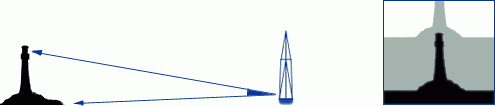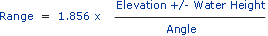• The angle in minutes total, thus 1° 12' = 72' total, and corrected for index error.
• Elevation in metres.
• Water height in metres above or below chart datum of object.
• Distance or Range in nautical miles.
• Ascertain whether the base of the object is beyond the horizon
• Corrected angle should be greater than 20'.
Though tables can be used for quick reference, this function is valid for objects higher than usually tabulated. An example with a lighthouse of 80 metres:
• Measured angle is 1° 19', index error is +6': angle = 73'.
• Let's assume water height at 3 metres above Mean Level datum.
• Range = 1.854*(80-3/73) = 1.96 nm.
The range can be used as a danger bearing.
Together with a compass bearing one object with known elevation results in a position fix. If more than one vertical sextant angle is combined the optimum angular spread should be maintained.
 Enter Angle (minutes total): Enter Elevation (metres): Distance is (nm):

Often, the correction for water height can be left out. Though, realizing that the horizon is closer than one might think, another correction is sometimes needed. In the Mediterranean Sea for example we can see mountain tops with bases lying well beyond the horizon. Mutatis mutandis, the structures, which they bear have bases beyond the horizon as well.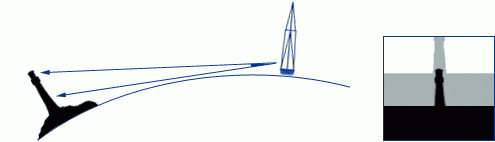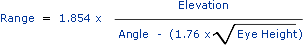This is the equation for finding the distance of an object of known elevation located beyond the horizon. In the denominator of this equation a compensating factor is included by which the measured angle should be reduced.

 Enter Eye Height (metres): Enter Angle (minutes total): Enter Elevation (metres): Distance is (nm):

Mathematics: vertical sextant angles

## Estimation of distance

The most obvious way to estimate distances is of course by using the distance between our eyes.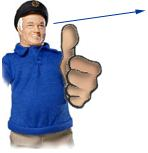If we sight over our thumb first with one eye then with the other, the thumb moves across the background, perhaps first crossing a tower second crossing a bridge.

The chart might tell that these structures are 300 m apart.
Use the ratio of: distance between eye and outstretched arm/distance between pupils: usually 10.
The objects are 3 kilometres away.
Other physical relationships are useful for quick reference. For example, one finger width held at arm's length covers about 2° arc, measured horizontally or vertically.
Two fingers cover 4°. Three fingers cover 6° and give rise to the three finger rule:
"An object that is three fingers high is about 10 times as far away as it is high."

## Estimation with horizon

The image on the right shows us that it is possible to estimate the height of any object that crosses the horizon as seen from our own point of view.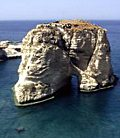This picture of the 'Pigeon Rocks' near Beirut harbour was taken from a crow's nest at a height of 34 metres.
The distance of the visible horizon (12 nm) is far larger than 34 metres. Therefore, we can - without any other information - estimate that these rocks have a height of 34 metres as well.

Factum: All tops crossing the horizon and with bases at sea level are on eye level.

Furthermore, if we see these rocks over a vertical angle of for example 7°= 0.1225 rad., then the range is 34/0.1225 = 277 metres.
Finally, plot both range and bearing in the chart to construct an EP, et Voilà!

## Fix by depth soundings

A series of depth soundings - in this example every 10 minutes - can greatly improve your position fix: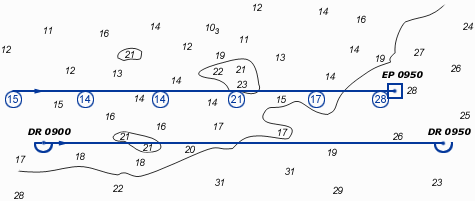• correct your soundings for tide, etc.;
• copy the DR course line on a transparent sheet;
• write the depths adjacent according to the times of the soundings;
• move the sheet over the chart to find its best location.
Due to leeway, currents or other factors the two course lines need not be parallel to or of same length as each other.

## Overview

• Line Of Position (LOP): The locus of points along which a ship's position must lie. A minimum of two LOP's are necessary to establish a fix. It is standard practice to use at least three LOP's when obtaining a fix, to guard against the possibility of and, in some cases, remove ambiguity.
• Range or Distance LOP: Obtained by using a stadimeter, sextant or radar. A circle equal in radius to the measured distance is plotted about the navigation aid; the ship must be somewhere on this circle.
• Running fix: A position determined by crossing lines of position obtained at different times and advanced or retired to a common time.
• Dead reckoning: Determining a position by plotting courses and speeds from a known position. It is also used to predict when lights become visible or to determine the set and drift of a current. DR positions are drawn in advance to prevent sailing into danger. A DR position will be plotted:
• every hour on the hour;
• at the time of every course change or speed change;
• for the time at which a (running) fix is obtained, also a new course line will be plotted;
• for the time at which a single LOP is obtained;
• and never draw a new course line from an EP position!
• Estimated position: The most probable position of a craft determined from incomplete data or data of questionable accuracy. Such a position might be determined by applying a correction to the dead reckoning position, as for estimated current; by plotting a line of soundings; or by plotting a LOP of questionable accuracy.
• Double angle on the bow: A method of obtaining a running fix by measuring the distance a vessel travels on a steady course while the relative bearing (right or left) of a fixed object doubles. The distance from the object at the time of the second bearing is equal to the run between bearings, neglecting drift.
• Four point fix: A special case of doubling the angle on the bow, in which the first bearing is 45° right or left of the bow. Due to angular spread this is the most precise isosceles fix.
• Special angle fix: A construction using special pairs of relative angles that give the distance travelled between bearings as equal to the navigation aids' range abeam.
• Distance from horizon: The distance measured along the line of sight from a position above the surface of the earth to the visible horizon.
• Sensible horizon: The circle of the celestial sphere formed by the intersection of the celestial sphere and a plane through the eye of the observer, and perpendicular to the zenith-nadir line.
• Visible horizon: The line where Earth and sky appear to meet. If there were no terrestrial refraction, visible and geometrical horizons would coincide. Also called : apparent horizon.
• Geometrical horizon: Originally, the celestial horizon; now more commonly the intersection of the celestial sphere and an infinite number of straight lines tangent to the earth's surface and radiating from the eye of the observer.
• Dipping range or Geographic range: The maximum distance at which the curvature of the earth and terrestrial refraction permit an aid to navigation to be seen from a particular height of eye (without regard to the luminous intensity of the light).
• Elevation: The height of the light above its chart datum in contrast to the height of the structure itself.
• Chart Datum: Officially: Chart Sounding Datum: An arbitrary reference plane to which both heights of tides and water depths are expressed on a chart. In the same chart heights can be related to other datums than depths.
• Vertical sextant angle: The method of using the subtended angle of a vertical object to find its range.
• Index error: In a marine sextant the index error is primarily due to lack of parallelism of the index mirror and the horizon glass at zero reading. A positive index error is subtracted and a negative index error is added.
• Estimation with horizon: Estimation of heights using the horizon: All tops crossing the horizon and with bases at sea level are on eye level.
• Estimation with depth effect: .
• Estimated position with soundings: .

Use the logo to navigate through this course,
...or go to the next chapter,
or download the complete navigation course as PDF-  including practice materials, exercises and answers.

E-mail:
E-mail:
Books·charts Sitemap| A-Z Contact RSS| Charterguide PDFs
2 February 2014
© 2000 – 2014 Diederik Willemsen |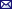Site map | A-Z index | Register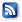|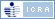|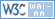|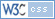|